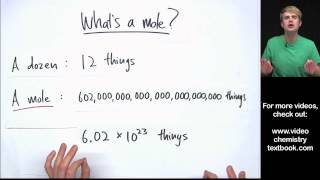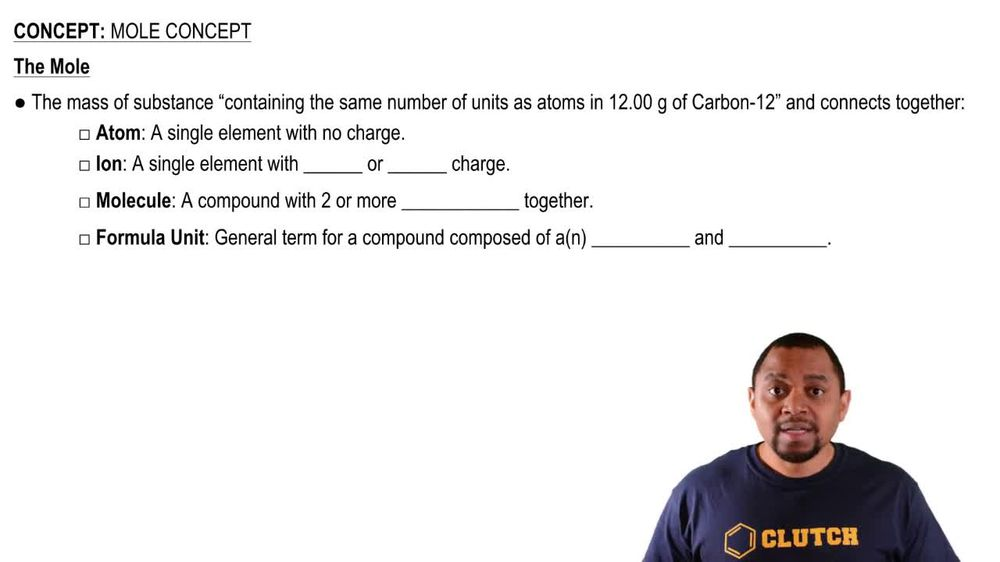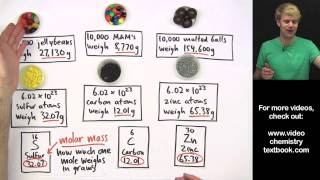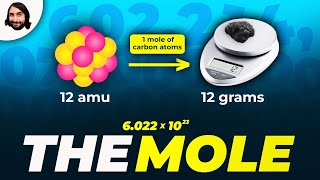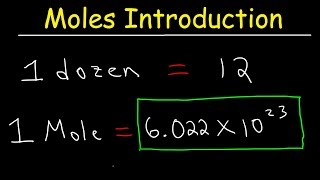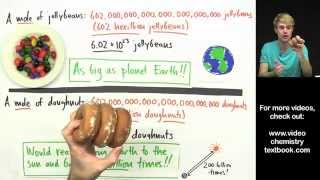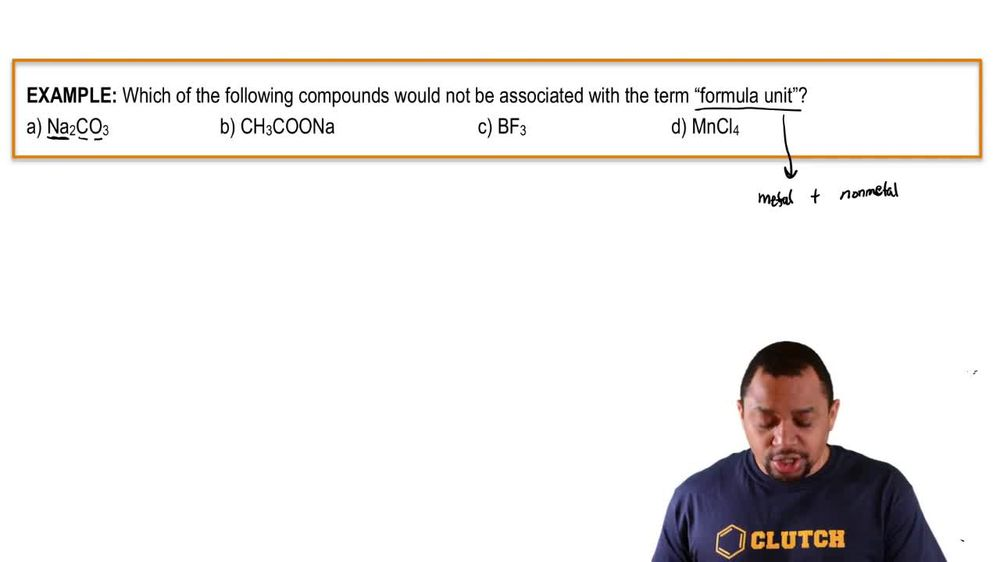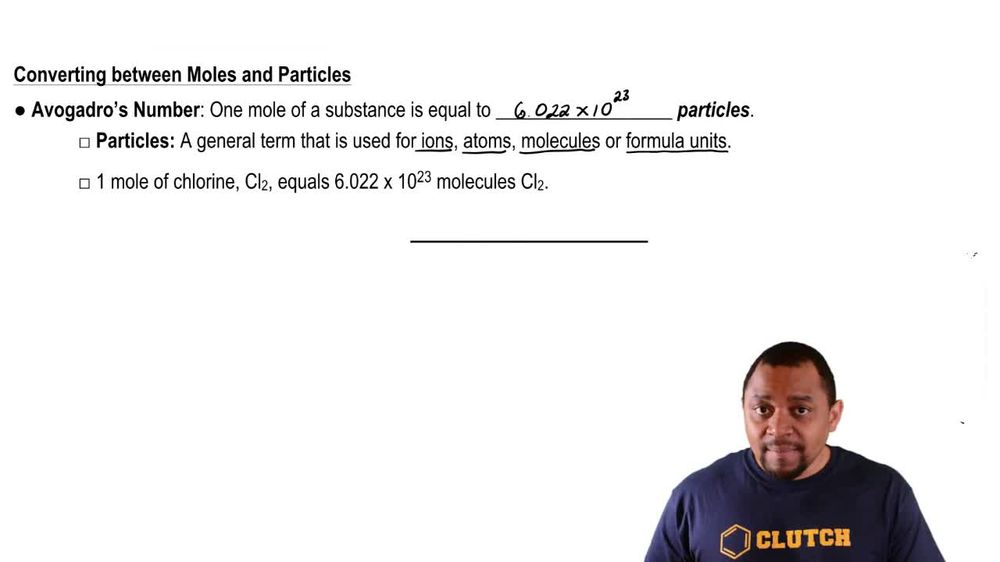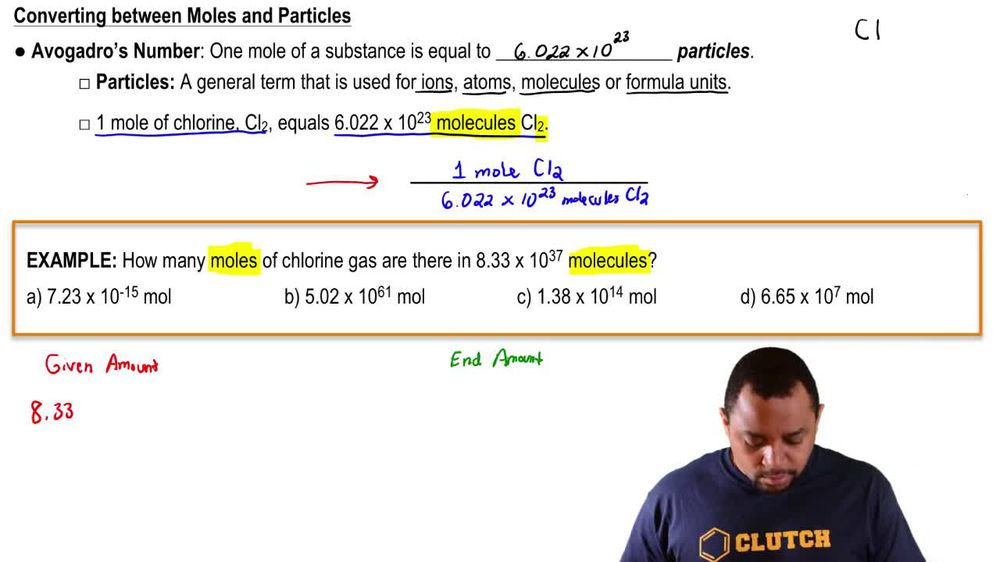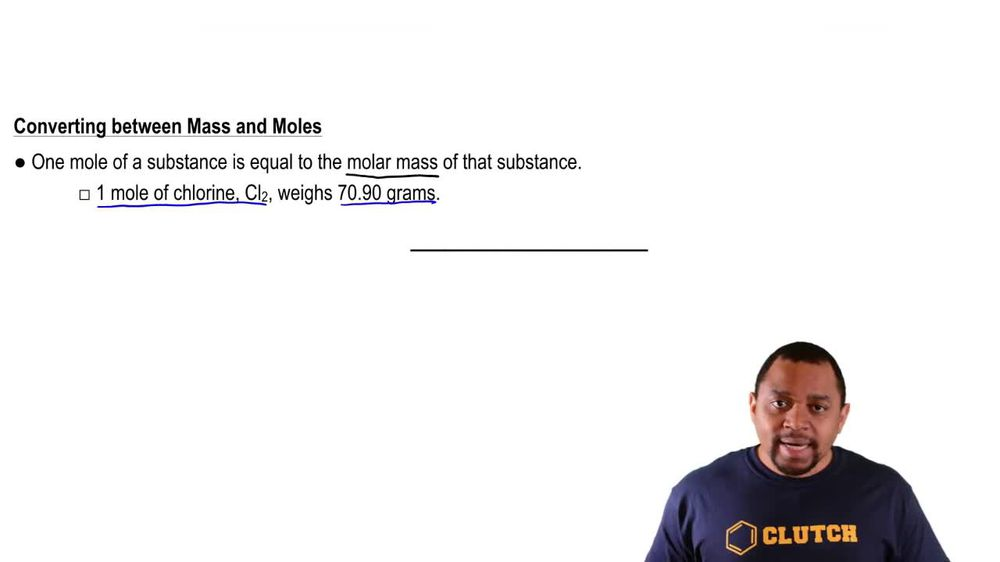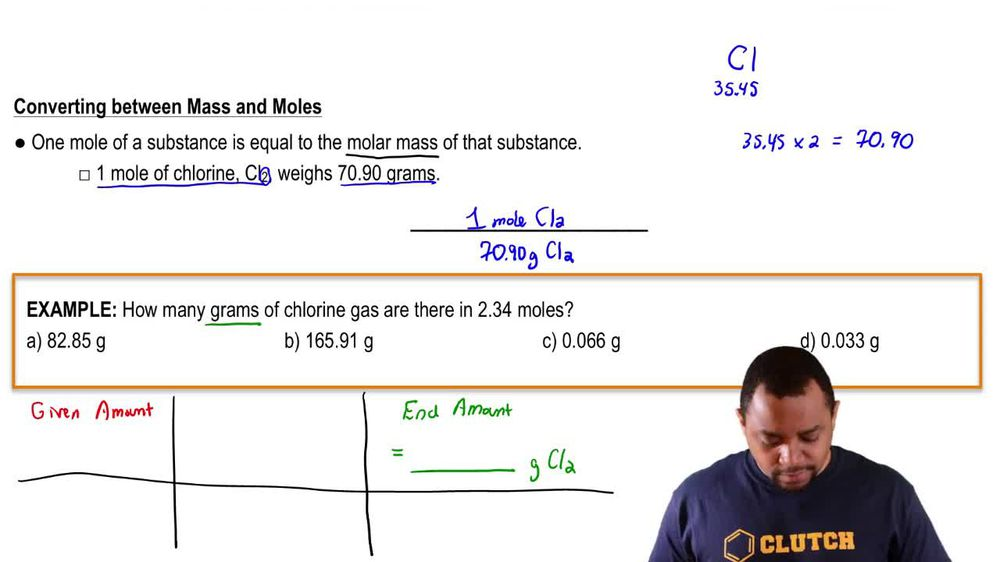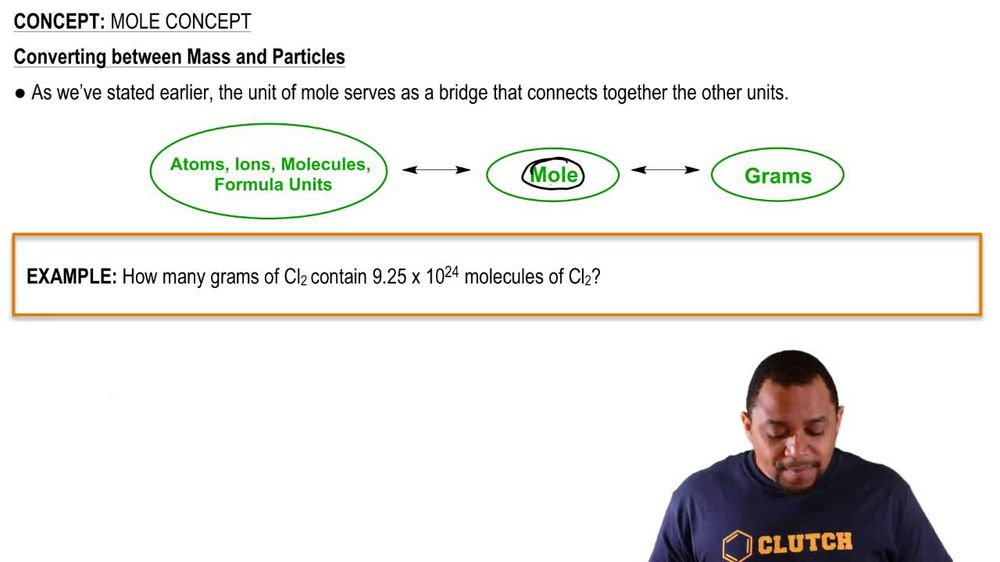Start typing, then use the up and down arrows to select an option from the list.
1. 2. Atoms & Elements2. Mole Concept
Problem

# Very small semiconductor crystals, composed of approximately 1000 to 10,000 atoms, are called quantum dots. Quantum dots made of the semiconductor CdSe are now being used in electronic reader and tablet displays because they emit light efficiently and in multiple colors, depending on dot size. The density of CdSe is 5.82 g/cm3. (b) CdSe quantum dots that are 2.5 nm in diameter emit blue light upon stimulation. Assuming that the dot is a perfect sphere and that the empty space in the dot can be neglected, calculate how many Cd atoms are in one quantum dot of this size.

Relevant Solution4m
Play a video:
Hi everyone here we have a question telling us. A fuel arena is an electro of carbon whose molecule consists of carbon atoms connected by a single and double bonds so as to form a close structure with fused rings of 5 to 7 atoms. Buckminster fuel marine is a type of fuel a ring with a molecular formula of C 60. The density of carbon 60 is 1.65 g per centimeters. Q Assuming a perfect sphere. That is 100 nm in diameter formed by this molecule such that the empty space between molecules can be neglected, calculate the approximate number of carbon atoms contained in one sphere of the size. So the volume of a sphere equals four thirds times pi times the radius cubed and the radius Equals the diameter divided by two. So our radius equals 100 nm divided by two Times 10 to the negative nine meters over one nanometer. And we want it in cm so times one cm Over 10 to the -2 m. And our nanometers are canceling out, our meters are canceling out, giving us centimeters and that equals five times 10 to the negative six centimeters. So the volume of the sphere equals four thirds pie, Times five times 10 to the negative six cm Cubed equals 5.23, 6 times 10 to the negative 16 cm cubed. So now we have to calculate the number of carbon atoms. So we need to calculate the molar mass of carbon 60. So we have 12.01, which is the molar mass of carbon times Equals .6 g per mole. So we have 5.236 times 10 to the negative 16 centimeters cubed Times 1.65 g Over one Centimeter Cube, which is our density times 60 moles of carbon over one more of carbon, 60 Times Alpha God was number 6.02, 2 times 10 to the 23rd Adams of carbon over one mold Carden and our centimeters cubed cancel out our grams cancel out and our molds cancel out, leaving us with atoms of carbon And that equals four times 10 to seventh Adams of carbon. And that is our final answer. Thank you for watching. Bye.